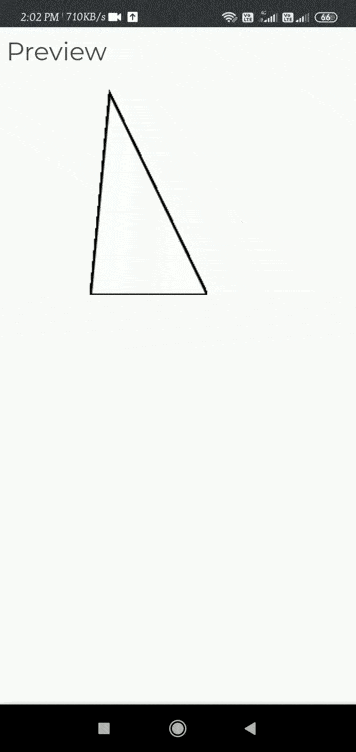Related Articles

# p5.js deviceTurned() Function

• Last Updated : 08 Apr, 2021

The function deviceTurned() s called when the device rotates by more than 90 degrees continuously. The axis that triggers the device turned() method is stored in the turnAxis variable.

This function can be locked to trigger on all three axis X, Y, or Z by comparing the turnAxis variable to ‘X’, ‘Y’ or ‘Z’.

This is Mobile application development function which allows access to specific sensors and modes of operation like detecting motion, acceleration, rotation, heading, and location in the devices.

Syntax:

`deviceTurned()`

Now we will run some examples in android phones.

• Step 1: Open online web editor of p5.js in the mobile phone using any browser “https://editor.p5js.org/
• Step 2: Write the following code in the editor section and run it to see the output.

Example 1:

## Javascript

 `// Run this example on a mobile device``// Rotate the device by 90 degrees in the``// X-axis or Y-axis or Z- axis to change the value .`` ` `let value = 0;``function` `draw() {``  ``fill(value);``  ``triangle(45, 100, 54, 5, 100, 100);``}`` ` `//  Set the the device turned function.``function` `deviceTurned() {``  ``if` `(turnAxis === ``'X'` `||turnAxis === ``'Y'``|| turnAxis === ``'Z'``) {``    ``if` `(value === 0) {``      ``value = 255;``    ``} ``else` `if` `(value === 255) {``      ``value = 0;``    ``}``  ``}``}`

Output: We will get this by turning our device by rotating in X,Y or  Z direction by 90 degree.My Personal Notes arrow_drop_up